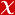## Stochastic discontinuous Galerkin methods with low–rank solvers for convection diffusion equations

Applied Numerical Mathematics, vol.172, pp.157-185, 2022 (Peer-Reviewed Journal)• Publication Type: Article / Article
• Volume: 172
• Publication Date: 2022
• Doi Number: 10.1016/j.apnum.2021.10.007
• Journal Name: Applied Numerical Mathematics
• Journal Indexes: Science Citation Index Expanded, Scopus, Academic Search Premier, Applied Science & Technology Source, Compendex, Computer & Applied Sciences, INSPEC, MathSciNet, zbMATH, DIALNET
• Page Numbers: pp.157-185
• Keywords: Uncertainty quantification, Stochastic discontinuous Galerkin, Error estimates, Low-rank approximations, Convection diffusion equation with random coefficients, PARTIAL-DIFFERENTIAL-EQUATIONS, FINITE-ELEMENT-METHOD, BI-CGSTAB, COEFFICIENTS, ALGORITHM, VARIANT, FLOW

#### Abstract

© 2021 IMACSWe investigate numerical behaviour of a convection diffusion equation with random coefficients by approximating statistical moments of the solution. Stochastic Galerkin approach, turning the original stochastic problem to a system of deterministic convection diffusion equations, is used to handle the stochastic domain in this study, whereas discontinuous Galerkin method is used to discretize spatial domain due to its local mass conservativity. A priori error estimates of the stationary problem and stability estimate of the unsteady model problem are derived in the energy norm. To address the curse of dimensionality of stochastic Galerkin method, we take advantage of the low–rank Krylov subspace methods, which reduce both the storage requirements and the computational complexity by exploiting a Kronecker–product structure of system matrices. The efficiency of the proposed methodology is illustrated by numerical experiments on the benchmark problems.